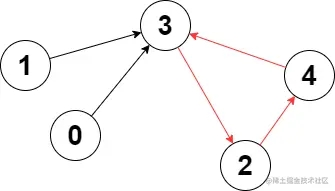﻿ C C++ 题解LeetCode2360图中的最长环示例_C 语言_脚本之家
C 语言# C C++ 题解LeetCode2360图中的最长环示例

## 题目描述## 具体实现

• 很容易想到每次都清空一遍标记数组，但是因为题目数据范围的原因，这样做是会超时的。

• 如果大于等于本轮开始节点的时间戳：说明是本轮遍历到新的一个环；
• 否则仅仅表示遍历到之前遍历过的节点，没有构成新的环（因为之前遍历过的节点如果有环也已经记录过了）

### 复杂度分析

• 时间复杂度：O(n)，其中 `n` 为 `edges` 的长度，也就是点的个数。
• 空间复杂度：O(n)。

## 代码实现

```class Solution {
public:
int longestCycle(vector<int>& edges) {
int n = edges.size();
// mp[i] = j 表示节点 i 是第 j 个遍历到的
int mp[n];
memset(mp, 0, sizeof(mp));
int ans = -1;
// k 进行计数
int k = 1;
for(int i = 0; i < n; i++){
// 从没有遍历过的点作为起点
if(mp[i] == 0){
int t = i;
// 找到第一个遍历过的节点
while(mp[t] == 0){
mp[t] = k++;
t = edges[t];
if(t == -1) break;
}
// 利用时间戳计算环的长度，取最大值
if(t != -1 && mp[t] >= mp[i]){
ans = max(ans, k - mp[t]);
}
}
}
return ans;
}
};
```

## 总结

• 该题的核心思想为记录每个节点被遍历到的时间戳，通过 时间戳来实现找新环的逻辑
• 因为是有向图且每个节点至多有一个出度，所以可以利用时间戳的方式来实现，需要注意这个前提条件。